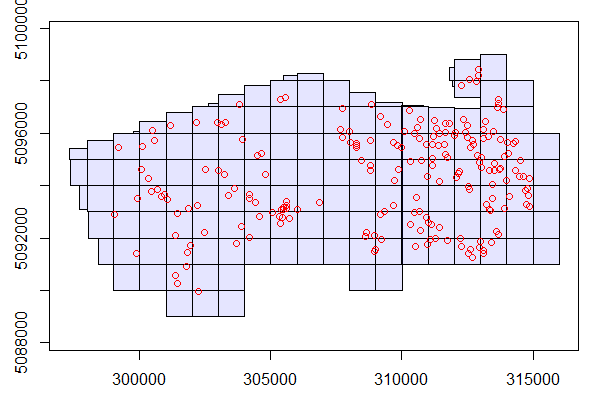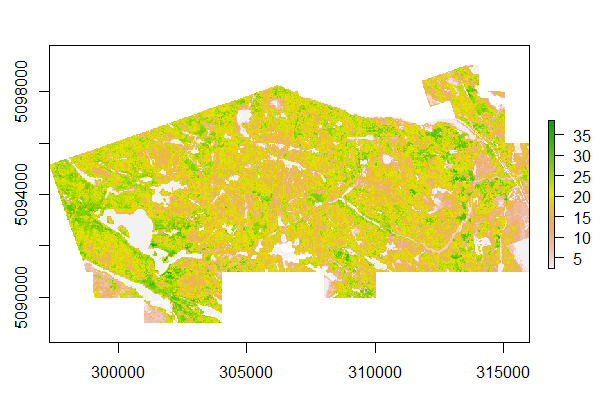# 16 The Area-Based Approach (ABA) to forest modelling

This section presents a complete workflow about processing ALS point cloud data to create wall-to-wall predictions of selected forest stand attributes using an area-based approach (ABA) to forest inventories. The steps used in this vignette as well as further details about enhanced forest inventories (EFI) are described in depth in White et al. 2013 and White et al. 2017. This vignette assumes that the user has a directory of classified and normalized `.las/.laz` files. First we load the package `sf` to work with spatial data.

``library(sf)``

The function `readLAScatalog()` seen in section 14 builds a `LAScatalog` object from a folder. Make sure the ALS files have associated index files - for details see: Speed-up the computations on a LAScatalog.

``````ctg <- readLAScatalog("folder/")
print(ctg)
#> class       : LAScatalog
#> extent      : 297317.5 , 316001 , 5089000 , 5099000 (xmin, xmax, ymin, ymax)
#> coord. ref. : +proj=utm +zone=18 +ellps=GRS80 +towgs84=0,0,0,0,0,0,0 +units=m +no_defs
#> area        : 125.19 km²
#> points      : 1.58 billion points
#> density     : 12.6 points/m²
#> num. files  : 138
plot(ctg)``````Now that our ALS data is prepared lets read in our inventory data using the `st_read()` function from the `sf` package. We read in a `.shp` file where each features is a point with a unique plot ID and corresponding plot level inventory summaries.

``plots <- st_read("PRF_plots.shp")``

Our `plots` object contains 203 plots with coordinates of plot centers, plot name, and stand attributes: Lorey’s height (HL), Basal area (BA), and gross timber volume (GTV).

``````plots
#> Simple feature collection with 203 features and 4 fields
#> geometry type:  POINT
#> dimension:      XY
#> bbox:           xmin: 299051.3 ymin: 5089952 xmax: 314849.4 ymax: 5098426
#> epsg (SRID):    NA
#> proj4string:    +proj=utm +zone=18 +ellps=GRS80 +units=m +no_defs
#> First 5 features:
#>    PlotID       HL       BA      GTV                 geometry
#> 1  PRF002 20.71166 38.21648 322.1351 POINT (313983.8 5094190)
#> 2  PRF003 19.46735 28.92692 251.1327 POINT (312218.6 5091995)
#> 3  PRF004 21.74877 45.16215 467.1033 POINT (311125.1 5092501)
#> 4  PRF005 27.76175 61.55561 783.9303 POINT (313425.2 5091836)
#> 5  PRF006 27.26387 39.78153 508.0337 POINT (313106.2 5091393)``````

To visualize where the plots are in our study area we can overlay them on the `LAScatalog`.

``````plot(ctg)
plot(plots, add = TRUE, col = "red")``````We have now prepared both our ALS and plot inventory data and can begin ABA processing.

## 16.2 ABA database building

To build a database ready for modeling the steps are the following:

1. Clip the regions of interest (stands) using a shapefile of stand locations.
2. Calculate derived metrics for each stand.
3. Associate stand attributes (ID,HL,BA,GTV) to the metrics.

This can be done with a single function `plot_metrics()`. We also use the `opt_filter()` function on the `LAScatalog` to ignore noise below 0 m at read time so that they do not influence future processing. We clip discs with a `radius` of 14.1 meters because our plot radius is 14.1 m.

``````opt_filter(ctg) <- "-drop_z_below 0" # Ignore points with elevations below 0

D <- plot_metrics(ctg, .stdmetrics_z, plots, radius = 14.1)``````

We have now calculated a variety of ALS metrics for each plot.

## 16.3 Statistical modeling

Here we provide a simple example of how to create an OLS model (`lm`) for Lorey’s Mean Height (HL). Using the `summary` and `plot` functions we found that the 85th percentile of height (`zq85`) for ALS metrics explain a large amount of variation in `HL` values. We are more than happy with this simple model.

``````m <- lm(HL ~ zq85, data = D)
summary(m)
#> Call:
#> lm(formula = HL ~ zq85, data = D)
#>
#> Residuals:
#>    Min     1Q Median     3Q    Max
#> -4.640 -1.053 -0.031  1.030  4.258
#>
#> Coefficients:
#>             Estimate Std. Error t value Pr(>|t|)
#> (Intercept)  2.29496    0.31116   7.376  4.2e-12 ***
#> zq85         0.93389    0.01525  61.237  < 2e-16 ***
#> ---
#> Signif. codes:  0 ‘***’ 0.001 ‘**’ 0.01 ‘*’ 0.05 ‘.’ 0.1 ‘ ’ 1
#>
#> Residual standard error: 1.5 on 201 degrees of freedom
#> Multiple R-squared:  0.9491, Adjusted R-squared:  0.9489
#> F-statistic:  3750 on 1 and 201 DF,  p-value: < 2.2e-16``````

Here we visualize the relationship between the observed (measured) and predicted (ALS-based) HL values.

``````plot(D\$HL, predict(m))
abline(0,1)``````## 16.4 Wall to wall modelling

Now that we have created a model using plot data, we need to apply that model across our entire study area.

To do so we first need to generate the same suite of ALS metrics (`.stdmetrics_z`) for our ALS data using the `pixel_metrics()` function on our original collection of files. Note that the `res` parameter is set to 25 because we want the resolution of our metrics to match the area of our sample plots (14.1 m radius = 625m2).

``metrics_w2w <- pixel_metrics(ctg, .stdmetrics_z, res = 25, pkg = "terra")``

To visualize any of the metrics you can use the `plot` function.

``````plot(metrics_w2w\$zq85)
plot(metrics_w2w\$zmean)
plot(metrics_w2w\$pzabovezmean)``````

## 16.5 Calculate wall-to-wall predictions

We can use the `predict()` function to produce `HL_pred` which is a wall-to-wall raster of predicted Lorey’s mean height.

``HL_pred <- predict(metrics_w2w, m)``

To visualize wall-to-wall predictions use

``plot(HL_pred)``